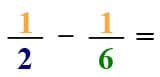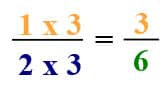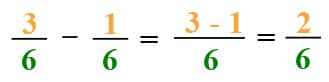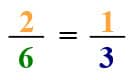The K5 Learning Blog urges parents to be pro-active in helping their children reach their full academic potential.

K5 Learning
provides free worksheets, workbooks and an online reading and math program for kindergarten to grade 5 students.# How to subtract fractions with unlike denominators

When the denominators in the fractions you want to subtract from each other are different, things get a little more complicated than subtracting fractions with like denominators.

Let’s say we want to subtract 1/6 from ½.## Find the least common multiple

First, we need to find the Least Common Multiple (LCM) for the denominators. Basically, we need to find a common multiple that they share.

The common multiples of 2 are 2, 4, 6, etc.
The common multiples of 6 are 6, 12, 18, etc.

The least common multiple is (3, 1) = 6.

That means in the first fraction we multiply the nominator and denominator by 3 to make the denominator 6 – equal to the denominator in the second fraction.

##Subtract the fractions

Now our fractions problem looks like this:

##Simplify the fraction

Now all we need to do is simplify the fraction. In an earlier blog about adding fractions, we described how to simplify fractions. If you need to review how to simplify fractions, you can read about that here.## Worksheets to practice subtracting fractions with different denominators

If you are looking to practice subtracting fractions with unlike denominators, check out our grade 5 fractions worksheets.